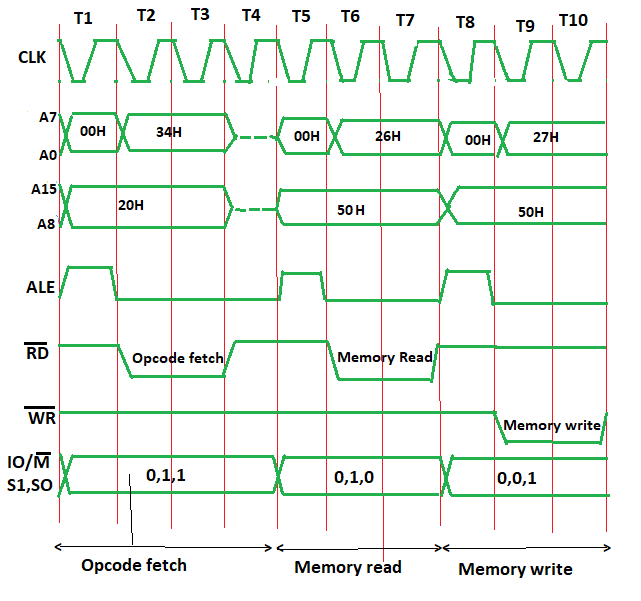# Timing diagram of INR M

• Last Updated : 26 Oct, 2018

Problem – Draw the timing diagram of the given instruction in 8085,

`INR M `

The content present in the designated register/memory location (M) is incremented by 1 and the result is stored in the same place. If the operand is a memory location, it is specified by the contents of HL pair.
Example:

```INR M
Opcode: INR
Operand: M ```

M is the memory location (say 5000H) and suppose the data present at M (or 5000H) is 26H, which is need to be incremented by 1. Hex code- 34H

Algorithm –
The instruction INR M is of 1 byte; therefore the complete instruction will be stored in a single memory address.
For example:

`2000: INR M `

The opcode fetch will be same as for other instructions in first 4 T states.
Only the Memory read and Memory Write need to be added in the successive T states.
For the opcode fetch the IO/M (low active) = 0, S1 = 1 and S0 = 1.
For the memory read the IO/M (low active) = 0, S1 = 1 and S0 = 0. Also, only 3 T states will be required.
For the memory write the IO/M (low active) = 0, S1 = 0 and S0 = 1 and 3 T states will be required.

The timing diagram of INR M instruction is shown below:In Opcode fetch ( t1-t4 T states ) –

• 00: lower bit of address where opcode is stored, i.e., 00
• 20: higher bit of address where opcode is stored, i.e., 20.
• ALE: provides signal for multiplexed address and data bus. Only in t1 it used as address bus to fetch lower bit of address otherwise it will be used as data bus.
• RD (low active): signal is 1 in t1 & t4 as no data is read by microprocessor. Signal is 0 in t2 & t3 because here the data is read by microprocessor.
• WR (low active): Signal is 1 throughout, no data is written by microprocessor.
• IO/M (low active): Signal is 0 in throughout because the operation is performing on memory.
• S0 and S1: both are 1 in case of opcode fetching.

In Memory read ( t5-t7 T states ) –

• 00: lower bit of address where opcode is stored, i.e, 00
• 50: higher bit of address where opcode is stored, i.e, 50.
• ALE: provides signal for multiplexed address and data bus. Only in t5 it used as address bus to fetch lower bit of address otherwise it will be used as data bus.
• RD (low active): signal is 1 in t5, no data is read by microprocessor. Signal is 0 in t6 & t7, data is read by microprocessor.
• WR (low active): signal is 1 throughout, no data is written by microprocessor.
• IO/M (low active): signal is 0 in throughout, operation is performing on memory.
• S0 and S1 – S1=1 and S0=0 for Read operation.

In Memory write ( t8-t10 T states ) –

• 00: lower bit of address where opcode is stored, i.e, 00
• 50: higher bit of address where opcode is stored, i.e, 50.
• ALE: provides signal for multiplexed address and data bus. Only in t8 it used as address bus to fetch lower bit of address otherwise it will be used as data bus.
• RD (low active): signal is 1 throughout, no data is read by microprocessor.
• WR (low active): signal is 1 in t8, no data is written by microprocessor. Signal is 0 in t9 & t10, data is written by microprocessor.
• IO/M (low active): signal is 0 in throughout, operation is performing on memory.
• S0 and S1 – S1=0 and S0=1 for write operation.
My Personal Notes arrow_drop_up